# Van der Waals Equation

We know that liquids are (almost) incompressible. So A(N,V,T) should diverge at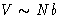. How can we arrange this?

Start from virial equation: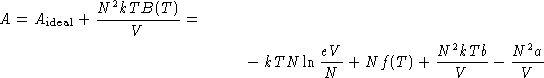Rearrange the terms: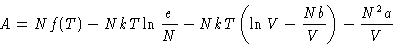(8)

We want an equation, that:

• Has the same behavior at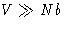• Diverges at.
Idea: substitute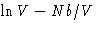by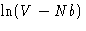.
• At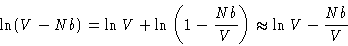• At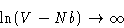Result: van der Waals formula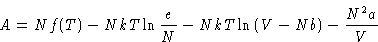(9)
Pressure: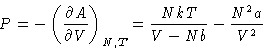At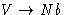it diverges.

© 1997 Boris Veytsman and Michael Kotelyanskii
Thu Sep 18 22:50:29 EDT 1997